## Difference of Squares

Recall that the product of conjugates produces a pattern called a difference of squares.

##### Example 1Factor x 2 – 16.

This polynomial results from the subtraction of two values that are each the square of some expression.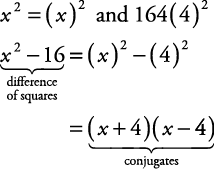##### Example 2

Factor 25 x 2 y 2 – 36 z 2.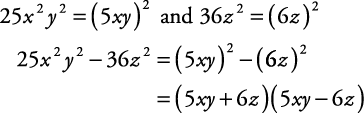##### Example 3

Factor ( a + b) 2 – ( cd) 2.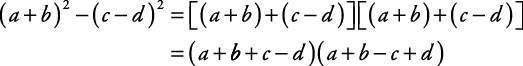##### Example 4

Factor y 2 + 9.

Even though y 2 and 9 are square numbers, the expression y 2 + 9 is not a difference of squares and is not factorable.

Many polynomials require more than one method of factoring to be completely factored into a product of polynomials. Because of this, a sequence of factoring methods must be used.

• First, try to factor by using the GCF.

• Second, try to factor by using the difference of squares.

##### Example 5

Factor 9 x 2 – 36.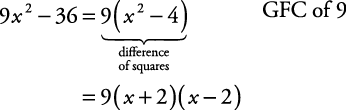##### Example 6

Factor 8( x + y) 2 – 18.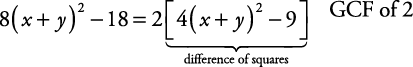Note: 4( x + y) 2 = [2( x + y)] 2 and 9 = 3 2.Back to Top
A18ACD436D5A3997E3DA2573E3FD792A